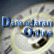##Dividend Regressions: January 2014

Variables used in the regression

1. Dividend Yield = Dividends per share in most recent year/ Current Stock Price
2. Dividend Payout Ratio = Dividends/ Net Income
3. Augmented Dividend Payout Ratio = (Dividends + Buybacks)/ Net Income
4. Beta: Regression or Bottom up beta
5. Expected Growth in EPS over next 5 years = Consensus analyst estimate (or your own) of expected growth in EPS . If you don't have an analyst estimate, use your own estimate of expected growth.
6. Market Debt to Capital = Debt/ (Debt + Market Value of Equity): If you have market value for debt, use it. If not, use book value of debt and market value of equity.

#### Dividend Yield Regression

 Model Summary Model R R Square Adjusted R Square Std. Error of the Estimate 1 .560a .314 .312 119.80360% a. Predictors: (Constant), Market Debt to Cap, Expected Growth in EPS (next 5 years), Regression Beta

 Coefficientsa,b Model Unstandardized Coefficients Standardized Coefficients t Sig. B Std. Error Beta 1 (Constant) 0.0383 .094 40.688 .000 Regression Beta -.0145 .00087 -.455 -16.628 .000 Expected Growth in EPS (next 5 years) -.0580 .006 -.266 -10.247 .000 Market Debt to Cap -.0120 .002 .184 6.667 .000 a. Dependent Variable: Dividend Yield b. Weighted Least Squares Regression - Weighted by Market Cap

• How do I use this regression?

Assume that you want to estimate the dividend yield for a firm with the following characteristics:

Expected growth in EPS = 10%

Regression beta = 1.20

Expected Growth in EPS over next 5 years = 12%

Market Debt to Capital = 20%

• Predicted Value

Expected Dividend yield = .0383 - 0.145 (1.20) - .058 (.12) - .012 (.20) = 1.15%

If your predicted value is less than zero, your predicted dividend yield is zero.

#### Augmented Dividend Payout Regression

 Model Summary Model R R Square Adjusted R Square Std. Error of the Estimate 1 .378a .143 .142 51.45390 a. Predictors: (Constant), Regression Beta, Expected Growth in EPS (next 5 years), Market Debt to Cap

 Coefficientsa,b Model Unstandardized Coefficients Standardized Coefficients t Sig. B Std. Error Beta 1 (Constant) .754 .037 20.646 .000 Expected Growth in EPS (next 5 years) -.007 .002 -.098 -4.176 .000 Market Debt to Cap .007 .001 .242 9.851 .000 Regression Beta -.460 .034 -.321 -13.521 .000 a. Dependent Variable: AdjPayoutratio b. Weighted Least Squares Regression - Weighted by Market Cap

• How do I use this regression?

Assume that you want to estimate the dividend payout ratio for a firm with the following characteristics:

Regression beta = 1.20

Expected Growth in EPS over next 5 years = 12%

Market Debt to Capital = 20%

• Predicted Value

Expected Dividend payout ratio= 0.754 - .007 (.12) +.007 *.20 - 0.460 (1.20) = .2026 or 20.26%

If your predicted value is less than zero, your predicted dividend payout ratio is zero.